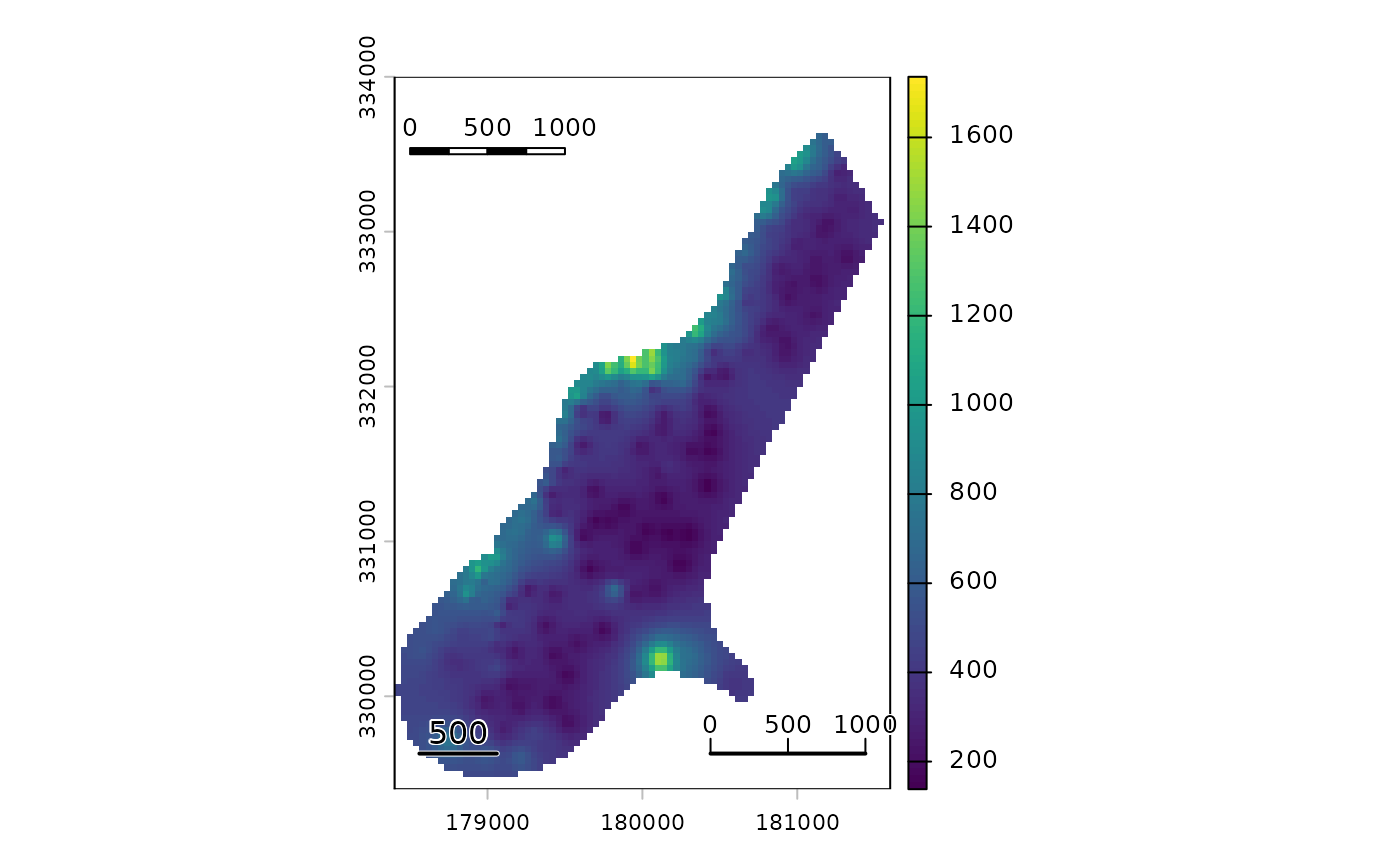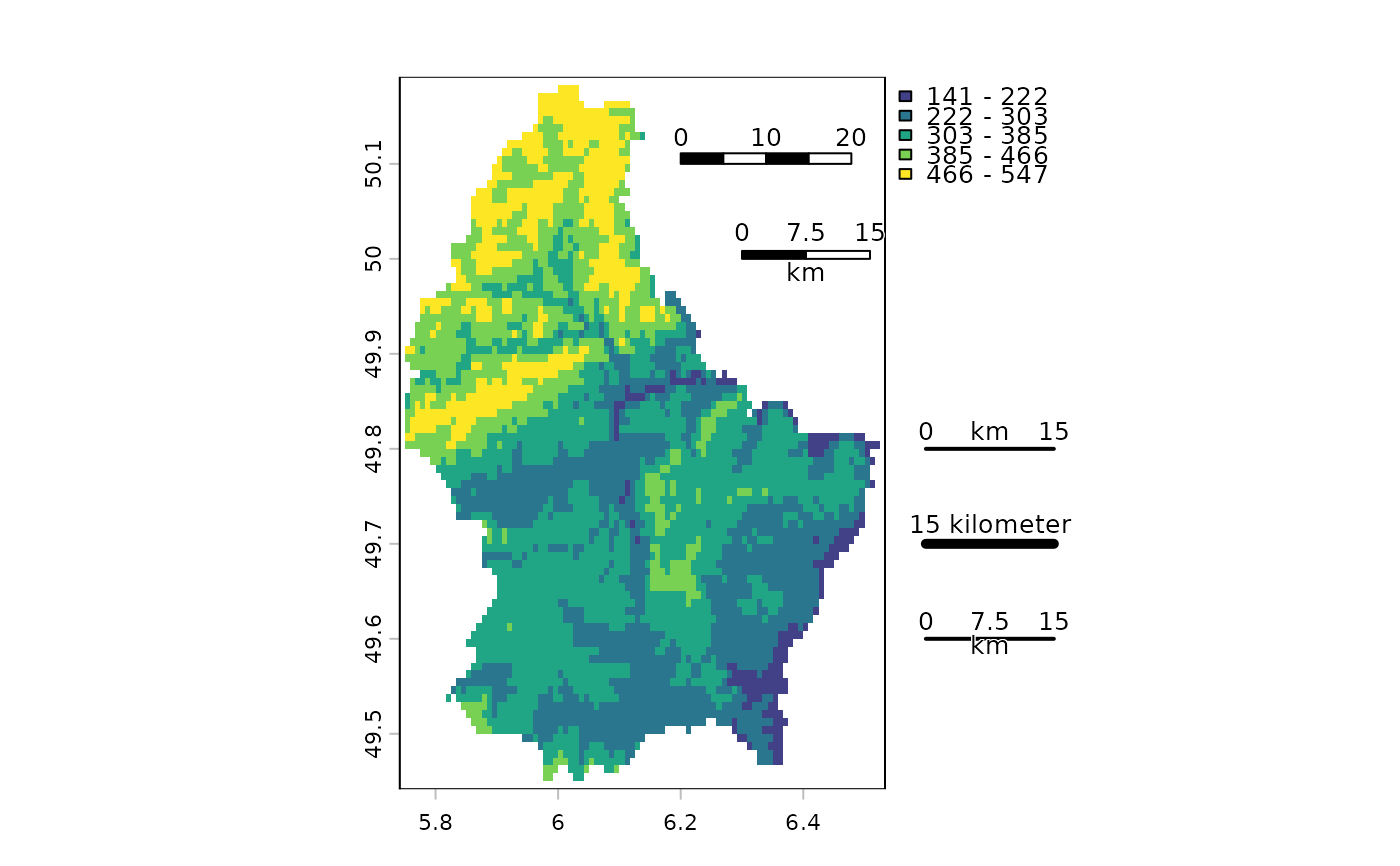Add a scale bar to a map

sbar(d, xy=NULL, type="line", divs=2, below="", lonlat=NULL, labels,
adj=c(0.5, -1), lwd=2, xpd=TRUE, ticks=FALSE, scaleby=1, halo=TRUE, ...)

## Arguments

d

numeric. Distance covered by the scale bar. For the scale bar, it should be in the units of the coordinates of the plot (map), and in km for angular (longitude/latitude) data; see argument lonlat. It can also be missing

xy

numeric. x and y coordinate to place the scale bar. It can also be one of following character values: "bottomleft", "bottom", "bottomright", topleft", "top", "topright", "left", "right", or NULL

type

for sbar: "line" or "bar"

divs

number of divisions for a bar: 2 or 4

below

character. Text to go below the scale bar (e.g., "kilometers")

lonlat

logical or NULL. If logical, TRUE indicates if the plot is using longitude/latitude coordinates. If NULL this is guessed from the plot's coordinates

labels

vector of three numbers to label the scale bar (beginning, midpoint, end)

lwd

line width for the "line" type of the scale bar

xpd

logical. If TRUE, the scale bar can be outside the plotting area

ticks

logical or numeric. If not FALSE, tick marks are added to a "line" scale bar. The length of the tick marks can be specified

scaleby

numeric. If labels is not provided. The labels are divided by this number. For example, use 1000 to go from m to km

halo

logical. If TRUE the "line" type scale bar gets a white background

...

graphical arguments to be passed to other methods

## Value

none

north, plot, inset

## Examples

f <- system.file("ex/meuse.tif", package="terra")
r <- rast(f)
plot(r)
sbar()
sbar(1000, xy=c(178500, 333500), type="bar", divs=4, cex=.8)
sbar(1000, xy="bottomright", divs=3, cex=.8, ticks=TRUE)
north(d=250, c(178550, 332500))f <- system.file("ex/elev.tif", package="terra")
r <- rast(f)
plot(r, type="interval")
sbar(20, c(6.2, 50.1), type="bar", cex=.8, divs=4)
sbar(15, c(6.3, 50), type="bar", below="km", label=c(0,7.5,15), cex=.8)
sbar(15, c(6.65, 49.8), cex=.8, label=c(0,"km",15))
north(type=2)
sbar(15, c(6.65, 49.7), cex=.8, label="15 kilometer", lwd=5)
sbar(15, c(6.65, 49.6), divs=4, cex=.8, below="km")